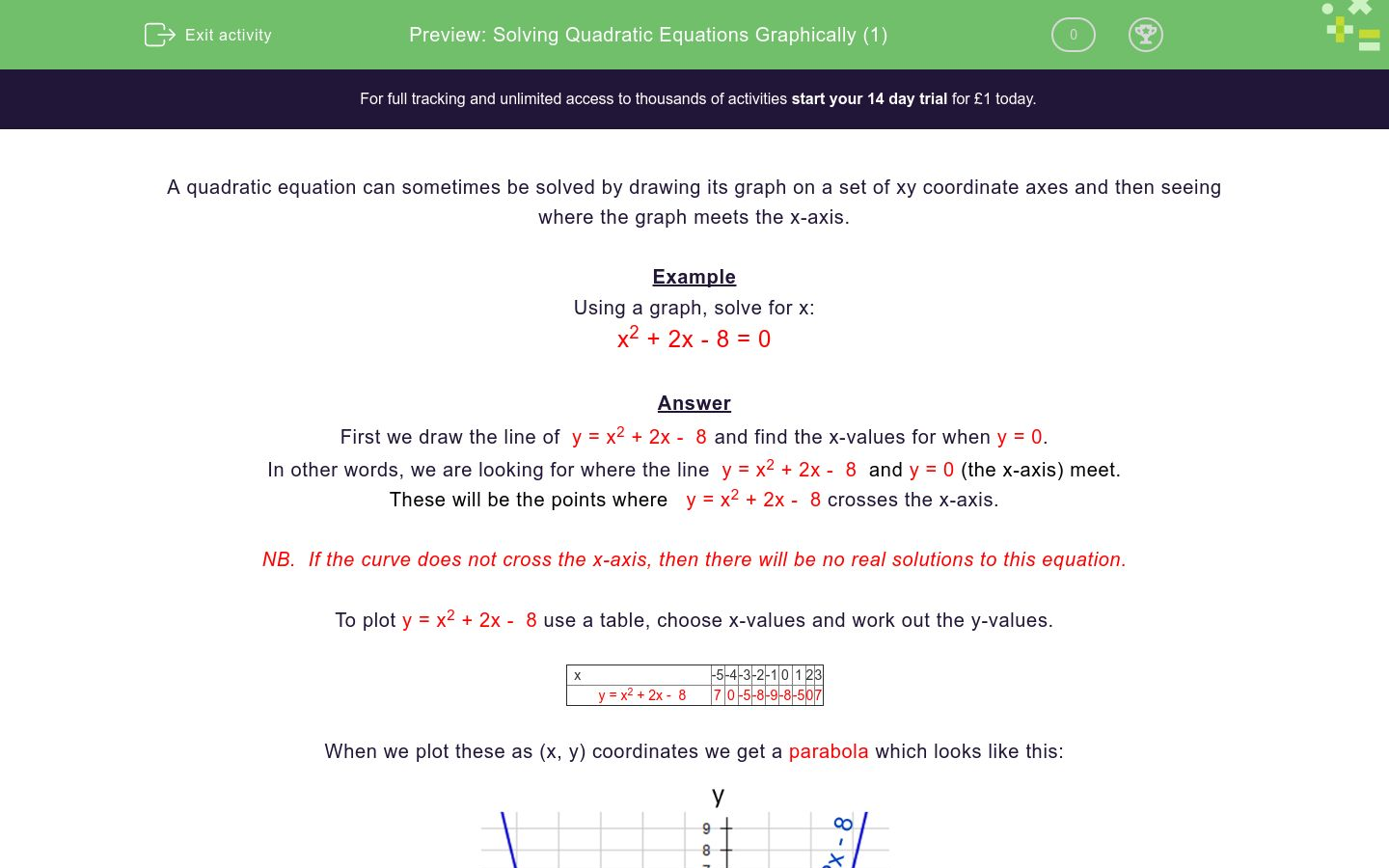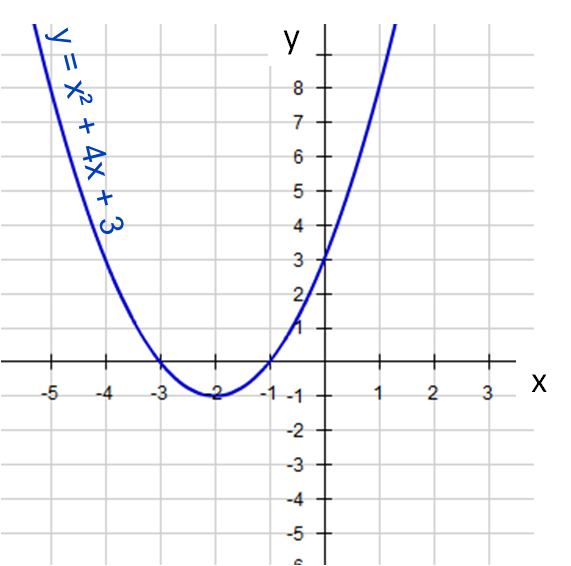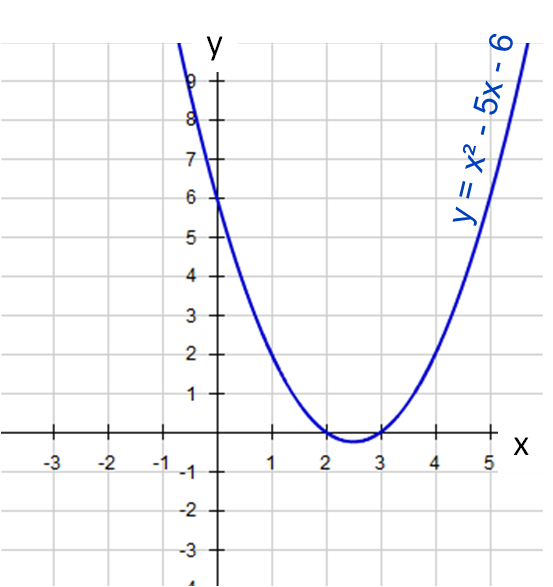# Solving Quadratic Equations Graphically (1)

In this worksheet, students solve quadratic equations by drawing graphs and finding the intercepts on the x-axis.Key stage:  KS 4

Curriculum topic:  Algebra

Curriculum subtopic:  Solve Quadratic Equations by Factorising

Difficulty level:### QUESTION 1 of 10

A quadratic equation can sometimes be solved by drawing its graph on a set of xy coordinate axes and then seeing where the graph meets the x-axis.

Example

Using a graph, solve for x:

x2 + 2x - 8 = 0

First we draw the line of  y = x2 + 2x -  8 and find the x-values for when y = 0.

In other words, we are looking for where the line  y = x2 + 2x -  8  and y = 0 (the x-axis) meet.

These will be the points where   y = x2 + 2x -  8 crosses the x-axis.

NB.  If the curve does not cross the x-axis, then there will be no real solutions to this equation.

To plot y = x2 + 2x -  8 use a table, choose x-values and work out the y-values.

 x -5 -4 -3 -2 -1 0 1 2 3 y = x2 + 2x -  8 7 0 -5 -8 -9 -8 -5 0 7

When we plot these as (x, y) coordinates we get a parabola which looks like this:The intercepts on the x-axis are at (-4, 0) and (2, 0)

Solution is:

x = -4 or x = 2

Using a graph, solve for x and select all the solutions to:

x2 + 2x = 0

x = ½

x = 0

x = -2

x = 2

Using a graph, solve for x and select all the solutions to:

x2 + 4x = 0

x = -4

x = -1

x = 0

x = 4

Using a graph, solve for x and select all the solutions to:

x2 + 4x + 3 = 0

x = -3

x = -1

x = 1

x = 3

Using a graph, solve for x and select all the solutions to:

x2 - 4x + 3 = 0

x = -3

x = -1

x = 1

x = 3

Using a graph, solve for x and select all the solutions to:

x2 - 2x - 3 = 0

x = -3

x = -1

x = 1

x = 3

Using a graph, solve for x and select all the solutions to:

x2 - 2x - 8 = 0

x = -4

x = -2

x = 4

x = 2

Using a graph, solve for x and select all the solutions to:

x2 - 5x + 6 = 0

x = -3

x = -2

x = 3

x = 2

Using a graph, solve for x and select all the solutions to:

-x2 + 4x = 0

x = 0

x = -4

x = 4

x = 2

Using a graph, solve for x and select all the solutions to:

-x2 - 5x = 0

x = 0

x = -5

x = 5

x = 25

Using a graph, solve for x and select all the solutions to:

-x2 + x + 6 = 0

x = -6

x = -2

x = 6

x = 3

• Question 1

Using a graph, solve for x and select all the solutions to:

x2 + 2x = 0

x = 0
x = -2
EDDIE SAYSThe intercepts on the x-axis are at (-2, 0) and (0, 0)

• Question 2

Using a graph, solve for x and select all the solutions to:

x2 + 4x = 0

x = -4
x = 0
EDDIE SAYSThe intercepts on the x-axis are at (-4, 0) and (0, 0)

• Question 3

Using a graph, solve for x and select all the solutions to:

x2 + 4x + 3 = 0

x = -3
x = -1
EDDIE SAYSThe intercepts on the x-axis are at (-3, 0) and (-1, 0)

• Question 4

Using a graph, solve for x and select all the solutions to:

x2 - 4x + 3 = 0

x = 1
x = 3
EDDIE SAYSThe intercepts on the x-axis are at (1, 0) and (3, 0)

• Question 5

Using a graph, solve for x and select all the solutions to:

x2 - 2x - 3 = 0

x = -1
x = 3
EDDIE SAYSThe intercepts on the x-axis are at (-1, 0) and (3, 0)

• Question 6

Using a graph, solve for x and select all the solutions to:

x2 - 2x - 8 = 0

x = -2
x = 4
EDDIE SAYSThe intercepts on the x-axis are at (-2, 0) and (4, 0)

• Question 7

Using a graph, solve for x and select all the solutions to:

x2 - 5x + 6 = 0

x = 3
x = 2
EDDIE SAYSThe intercepts on the x-axis are at (2, 0) and (3, 0)

• Question 8

Using a graph, solve for x and select all the solutions to:

-x2 + 4x = 0

x = 0
x = 4
EDDIE SAYSThe intercepts on the x-axis are at (0, 0) and (4, 0)

• Question 9

Using a graph, solve for x and select all the solutions to:

-x2 - 5x = 0

x = 0
x = -5
EDDIE SAYSThe intercepts on the x-axis are at (-5, 0) and (0, 0)

• Question 10

Using a graph, solve for x and select all the solutions to:

-x2 + x + 6 = 0

x = -2
x = 3
EDDIE SAYSThe intercepts on the x-axis are at (-2, 0) and (3, 0)

---- OR ----

Sign up for a £1 trial so you can track and measure your child's progress on this activity.

### What is EdPlace?

We're your National Curriculum aligned online education content provider helping each child succeed in English, maths and science from year 1 to GCSE. With an EdPlace account you’ll be able to track and measure progress, helping each child achieve their best. We build confidence and attainment by personalising each child’s learning at a level that suits them.